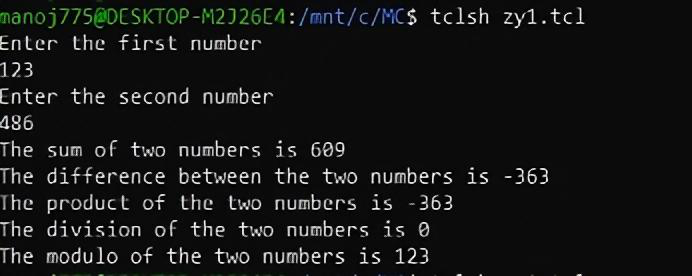# TCL script to demonstrate procedures

• Difficulty Level : Medium
• Last Updated : 02 Jun, 2021

In this article, we will know how to use procedures in TCL. Procedures are just like functions we use in any other programming language such as C, Java, Python, etc. Just like functions, procedures take arguments and return some value. Let’s go through a simple program that calls procedures to print add, subtract, multiply, divide, and modulo two numbers step-by-step.

Pre-requisite –
If you want to know the basics of TCL script then kindly go through this article https://www.geeksforgeeks.org/basics-of-ns2-and-otcltcl-script/.

Overview:
We will try to understand the syntax of a procedure in TCL by exploring the code block-by-block. Furthermore, we will also look at the syntax for procedures in C programming to compare with and understand better.

TCL script to demonstrate procedures :
Let’s discuss the following steps as follows.

Step-1 :
Let’s first define our procedures. We do that using the proc keyword. The procedures addnumbers {}, sub numbers {}, mulnumbers {}, divnumbers {} and modnumbers {} are created to compute the sum, difference, product, division, and modulo of two numbers respectively.

```TCL script -

proc addnumbers { a b } {
return [expr \$a + \$b]
}

//Subtraction
proc subnumbers { a b } {
return [expr \$a - \$b]
}

//Multiplication
proc mulnumbers { a b } {
return [expr \$a * \$b]
}

//Division
proc divnumbers { a b } {
return [expr \$a / \$b]
}

//Modulus
proc modnumbers { a b } {
return [expr \$a % \$b]
}```

As shown above, the syntax can be generalized as follows.

```proc procedurename {arguments} {
#body of the procedure
}```

Note –
The syntax has to be exactly as shown above. If you neglect the spaces or type the opening curly brace in a new line, the result will be an error. The same syntax is followed by all conditional statements in TCL. Also note that procedures like functions, may or may not have a return type. Now let’s look at how the above set of functions would look like in C programming.

## C

 `//Addition``int` `addnumbers(``int` `a, ``int` `b) {``               ``return` `a + b; }` `//Subtraction``int` `subnumbers(``int` `a, ``int` `b) {``               ``return` `a - b; }` `//Multiplication``int` `mulnumbers(``int` `a, ``int` `b) {``               ``return` `a * b; }` `//Division``float` `divnumbers(``float` `a, ``float` `b) {``               ``return` `a / b; }` `//Modulus``int` `modnumbers(``int` `a, ``int` `b) {``               ``return` `a % b; }`

Step-2 :
The next step is to read two numbers a and b using gets.

```puts "Enter the first number"
gets stdin a
puts "Enter the second number"
gets stdin b```

Step-3 :
The final step is to print all the required values. Here, we will also look at the syntax in C programming to understand how we call a function in TCL.

```puts "The sum of two numbers is [addnumbers \$a \$b]"
puts "The difference between the two numbers is [subnumbers \$a \$b]"
puts "The product of the two numbers is [subnumbers \$a \$b]"
puts "The division of the two numbers is [divnumbers \$a \$b]"
puts "The modulo of the two numbers is [modnumbers \$a \$b]"```

So, we saw above the syntax to call a procedure would look like the following.

`[procedurename \$argument1 \$argument2 .....]`

Now let’s compare with the syntax in C programming to call a function.

`functionname(argument1,argument2,.....)`

Step-4 :
Finally, we view the entire code with the output as follows.

Code –

```//Addition
return [expr \$a+\$b]
}

//Subtraction
proc subnumbers {a b} {
return [expr \$a-\$b]
}

//Multiplication
proc mulnumbers {a b} {
return [expr \$a*\$b]
}

//Division
proc divnumbers {a b} {
return [expr \$a/\$b]
}

//Modulus
proc modnumbers {a b} {
return [expr \$a%\$b]
}

//Input-1
puts "Enter the first number"
gets stdin a

//Input-2
puts "Enter the second number"
gets stdin b

//called procedures
puts "The sum of two numbers is [addnumbers \$a \$b]"
puts "The difference between the two numbers is [subnumbers \$a \$b]"
puts "The product of the two numbers is [subnumbers \$a \$b]"
puts "The division of the two numbers is [divnumbers \$a \$b]"
puts "The modulo of the two numbers is [modnumbers \$a \$b]"```

Output :Output for a=123 and b=486

My Personal Notes arrow_drop_up